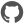# Random

Namespace: FsCheck

Generate random numbers based on splitting seeds. Based Hugs' Random implementation.

## Nested types and modules

 Type Description StdGen

### Functions and values

 Function or value Description ``` a1 ``` Signature: int``` a2 ``` Signature: int``` divMod64 n d ``` Signature: n:int64 -> d:int64 -> int64 * int64``` hMod64 n d ``` Signature: n:int64 -> d:int64 -> int64``` m1 ``` Signature: int``` m2 ``` Signature: int``` mkStdGen(s) ``` Signature: s:int64 -> StdGen``` newSeed() ``` Signature: unit -> StdGen``` q1 ``` Signature: int``` q2 ``` Signature: int``` r1 ``` Signature: int``` r2 ``` Signature: int``` range ``` Signature: int * int -> StdGen -> int * StdGen``` split ``` Signature: StdGen -> StdGen * StdGen``` stdNext(arg1) ``` Signature: StdGen -> int * StdGen``` stdRange(l, h) rng ``` Signature: (l:int * h:int) -> rng:StdGen -> int * StdGen``` stdSplit(arg1) ``` Signature: StdGen -> StdGen * StdGen